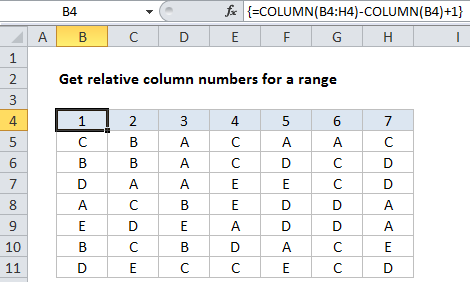## Excel Office

Excel How Tos, Tutorials, Tips & Tricks, Shortcuts

# How to get relative column numbers in a range in Excel

This tutorial shows how to get a full set of relative column numbers in a range using an array formula based on the COLUMN function.

## Formula

`{=COLUMN(range)-COLUMN(range.firstcell)+1}`On the worksheet, this must be entered as multi-cell array formula using Control + Shift + Enter

Worked Example:   How to get address of first cell in range in Excel

This is a robust formula that will continue to generate relative numbers even when columns are inserted in front of the range.

## Explanation

In the example shown, the array formula in B4:H4 is:

`{=COLUMN(B4:H4)-COLUMN(B4)+1}`

### How this formula works

The first COLUMN function generates an array of 7 numbers like this:

Worked Example:   How to use Excel COLUMN Function

{2,3,4,5,6,7,8}

The second COLUMN function generates an array with just one item like this:

{2}

which is then subtracted from the first array to yield:

{0,1,2,3,4,5,6}

Finally, 1 is added to get:

Worked Example:   Check if multiple cells have same value in Excel

{1,2,3,4,5,6,7}

### With a named range

You can adapt this formula to use with a named range. For example, in the above example, if you created a named range “data” for B4:H4, you can use this formula to generate column numbers:

`{=COLUMN(data)-COLUMN(INDEX(data,1,1))+1}`